Paul Yates overcomes some common misconceptions and misunderstandings encountered when dealing with fractionsAn experienced mathematician would have no difficulty in looking at an expression such as:

3 + 4 x 2 - 1

and assigning it a value of 10. This comes from multiplying 4 and 2, adding 3 then subtracting 1. However, the less experienced might reasonably add 3 to 4 to give 7, and multiply this by 1 (obtained from 2 - 1) to give 7.

To obtain the correct value one needs to be aware of the precedence rules which govern the order in which we apply the operators with which the numbers are combined within the expression.

### BIDMAS

Such rules are often remembered using the mnemonic BIDMAS, which stands for Brackets, Indices, Division, Multiplication, Addition and Subtraction. Applying this to the expression above, we perform the multiplication first to give:

3 + 8 -1

11 – 1

and finally the subtraction to give the final value of 10. Note that brackets can be used to change this order. If they were included to make the original expression:

(3 + 4) x (2 – 1)

We perform the operations within brackets first to give:

7 x 1

which has the value 7 as we saw above.

### A more complex example

A more complicated expression will show how the rules also deal with indices and division. ConsiderWe begin by evaluating the quantity within brackets first. As this consists of several steps we also have to apply the rules to the expression within the brackets. There is a further complication in this example in that the fractional quantity also contains what we might consider as implied brackets. It is often helpful to include such implied brackets explicitly, so this could be written as:So we need to evaluate the numerator and denominator of the fraction separately.

Looking at the numerator first, we identify any indices, and see that:

23 = 8

We can now perform the subtraction:

24 – 8 = 16

The denominator consists of the simple summation:

3 + 1 = 4

Returning to the complete original expression we now have: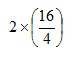Evaluating the bracket gives:and so the overall expression is reduced to:

2 x 4 = 8

### Teaching Issues

Tall and Razali1 hypothesised that less able students see the symbols in an expression as a process to be carried out in the written order, while more able students can chunk the symbols together according to given conventions. They also note that the former approach is the way that a calculation is carried out on some calculators.

Radcliffe has outlined a number of misunderstandings which may arise when applying the rule outlined above.

1. Once brackets have been identified, the rule needs to be applied to the operators within each bracket. Simplifying inside the bracket is not the same as eliminating the bracket.
2. Fractions are often written to imply that the terms within the numerator and within the denominator should be grouped together, as in the example above. I tend to think of these as implied brackets, which it is often beneficial to show explicitly.
3. Despite the use of the BIDMAS mnemonic, multiplication and division have equal priority, as do addition and subtraction. This can allow for efficiencies in computation when the rule does not need to be applied in six (or more) distinct stages.

### Examples

The following three examples will illustrate the correct application of operators that occur in mathematical expressions within chemistry.

#### 1. Calculation of relative atomic mass of chlorine2

This expression is based on the atomic masses and relative abundances of the individual isotopes of chlorine.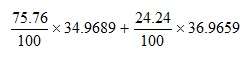Applying the precedence rules show that we need to perform the multiplications first to give:Which is evaluated as 35.45 using the rules for quoting an appropriate number of significant figures and treating 100 as an exact integer.

#### 2. The mole fraction of a solute in a solution3 can be expressed aswhere w is the mass of solute, m is the molecular mass of solute, W the mass of solvent and M the molecular mass of solvent.

Consider a solution of sodium chloride formed by dissolving 10.0 g of solute in 100g of water. Substituting quantities into the equation gives:I would suggest that a useful first stage would be to include the implied brackets so that it reads: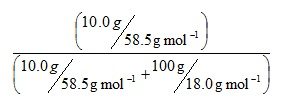This directs us to evaluate the brackets first, and both can be evaluated in parallel. Our rules instruct us to perform the divisions before the addition, so we have: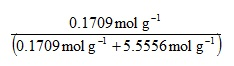Now evaluating the denominator bracket gives:

0.1709 mol g-1 + 5.5556 mol g-1 = 5.7265 mol g-1

So the overall expression is: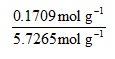and the mole fraction is evaluated as 0.0298.

#### 3. For the reaction

PCl5(g) = PCl3(g) + Cl2(g)

the equilibrium constant Kp can be defined as: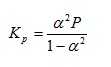where a is the degree of dissociation and P is the total pressure of the system. If we wish to evaluate Kp when P = 4.38 x 105 Pa and a = 0.250, it may again be helpful to begin by including implied brackets to give:As before, on the grounds of efficiency we can evaluate each bracket in parallel. Our precedence rules direct us to evaluate the indices first.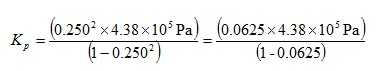We can now perform the multiplications in the top bracket and the subtraction in the lower bracket to give: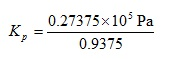We can now perform the final multiplication and division to give:

Kp = 29200 Pa

### History of Operations

This has been investigated by Peterson, who notes however that it has not been possible to obtain any definitive answers. The developmental nature of the rules is emphasised by noting that there is still current development in this area, such as in the discussion of whether implicit multiplication (e.g. 2a) should take precedence over explicit multiplication (2 x x) or division (2/x).

Paul Yates is the discipline lead in the physical sciences at the Higher Education Academy and is the author of the book ‘Chemical Calculations’.